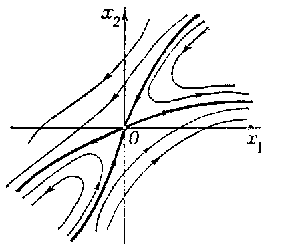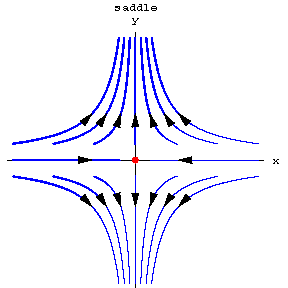##### Actions

(diff) ← Older revision | Latest revision (diff) | Newer revision → (diff)

A type of arrangement of the trajectories of an autonomous system of planar ordinary differential equations

$$\tag{* } \dot{x} = f ( x),\ \ x \in \mathbf R ^ {2} ,\ \ f: G \subset \mathbf R ^ {2} \rightarrow \mathbf R ^ {2} ,$$

$f \in C ( G)$, where $G$ is the domain of uniqueness, in a neighbourhood of a singular point (equilibrium position) $x _ {0}$. This type is characterized as follows. There is a neighbourhood $U$ of $x _ {0}$ such that for all trajectories of the system that start in $U \setminus \{ x _ {0} \}$, both the positive and negative semi-trajectories are diverging (as time passes they leave any compact set $V \subset U$). The exceptions are four trajectories, called the separatrices of the saddle. For two of these, the negative semi-trajectories are diverging and the positive semi-trajectories converge to $x _ {0}$, and for the other two the situation is just the opposite. The first two separatrices are called stable, and the second two, unstable. The stable separatrices, completed by $x _ {0}$, form a smooth curve through $x _ {0}$— the stable manifold of the saddle. The unstable separatrices, together with $x _ {0}$, form the smooth unstable manifold of the saddle. The point $x _ {0}$ is also called the saddle.

The saddle $x _ {0}$ is unstable in the sense of Lyapunov (cf. Lyapunov stability). Its Poincaré index is $- 1$( cf. Singular point). For a system (*) of class $C ^ {1}$( $f \in C ^ {1} ( G)$) with a non-zero matrix $A = f ^ { \prime } ( x _ {0} )$, a stationary point $x _ {0}$ is a saddle when the eigenvalues $\lambda _ {1} , \lambda _ {2}$ of $A$ satisfy the condition $\lambda _ {1} \lambda _ {2} < 0$( a simple saddle; see Fig. a, where $x _ {0} = 0$) but it can also be a saddle when $\lambda _ {1} = 0 \neq \lambda _ {2}$, or $\lambda _ {1} = \lambda _ {2} = 0$.Figure: s083020a

In any of these cases the separatrices of the saddle are tangential at $x _ {0}$ to the directions defined by the eigenvectors of $A$.

If the system (*) is linear ( $f ( x) = A ( x - x _ {0} )$, where $A$ is a constant matrix with eigenvalues $\lambda _ {1}$ and $\lambda _ {2}$), then a point $x _ {0}$ of it is a saddle only when $\lambda _ {1} \lambda _ {2} < 0$. In this case the separatrices of the saddle $x _ {0}$ are rectilinear, and all the other trajectories (distinct from $x _ {0}$) are affine images of the hyperbolas of the form $x _ {2} = c | x _ {1} | ^ {\lambda _ {2} / \lambda _ {1} }$, $c \in \mathbf R \setminus \{ 0 \}$( Fig. b).Figure: s083020b

The term "saddle" is also used for any of the arrangements of the trajectories of the system (*) in a neighbourhood $U$ of an isolated stationary point $x _ {0}$, where only a finite number $m$( $\geq 2$) of trajectories in $U \setminus \{ 0 \}$ approaches $x _ {0}$ and where each of them, completed by $x _ {0}$, touches at this point in a definite direction (an $m$- separatrix saddle). Certain types of stationary points of autonomous systems of ordinary differential equations of order $n \geq 3$ are also called saddles. For references, see Singular point of a differential equation.